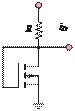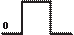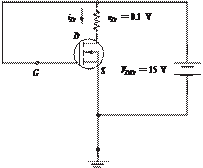### Create an Account

Home / Questions / In the circuit shown in Figure P114 the pchannel transistor has VT 2 V and K

# In the circuit shown in Figure P114 the pchannel transistor has VT 2 V and K

In the circuit shown in Figure P11.4, the p-channel transistor has V= 2 V and = 10 mA/V2. Find and vfor i= 0.4 mA.+20 VvG                5 V

Figure P11.9

11.10 In the circuit shown in Figure P11.10, a drain voltage of 0.1 V is established. Find the current ifor VT  = 1 V and = 0.5 mA/V2.Figure P11.4

Jun 18 2020 View more View LessSubscribe To Get Solution